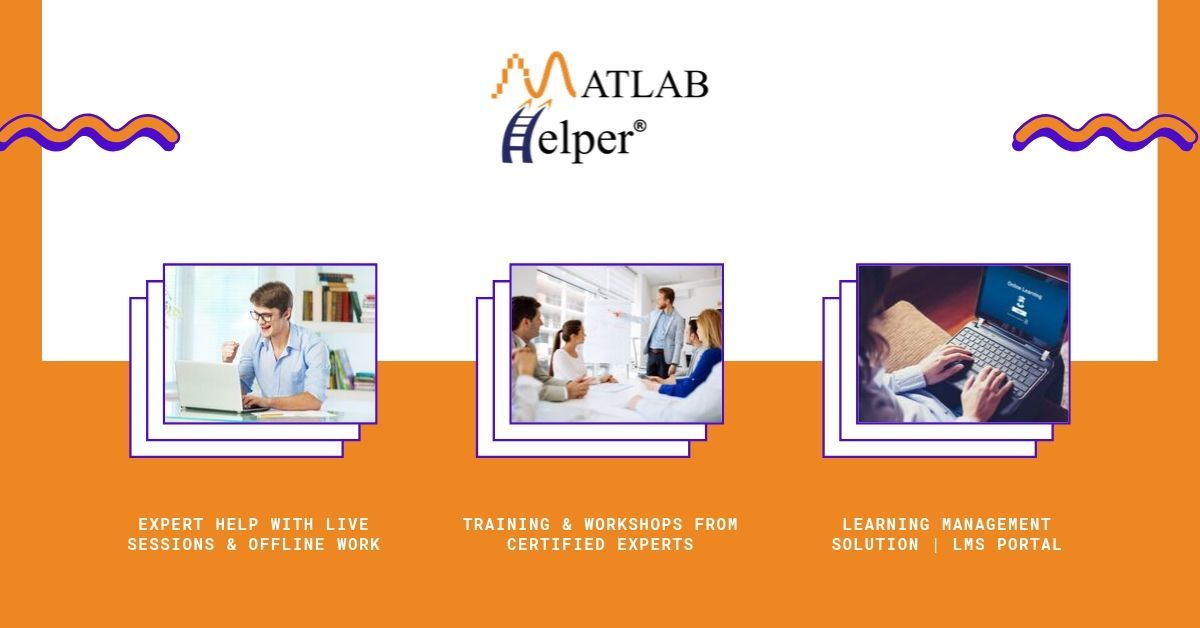# Decode Encoded Instruction in MATLAB

January 28, 2022

By  Sneha

lang: en_US

You may be curious to know how encoded information returns to its original form and internal working of the same. The method used to convert the encoded information to the original form is called "Decoding" and the chip used is known as "Decoder". Let's understand the technique/logic behind decoding.

## Introduction:

A Binary Decoder is a combinational logic circuit, a type of digital logic implemented by Boolean circuits, that converts binary information from the n coded inputs to a maximum of 2n unique outputs. That means it has n input lines and 2n output lines. We represent the Decoder symbolically with its size as n:2n Decoder.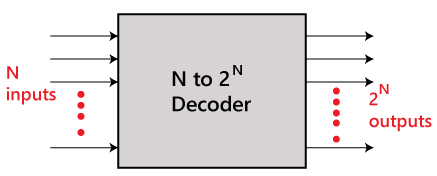Decoder Block Diagram

It converts every unique combination of input states to a specific combination of output states. In addition to integer data inputs, some decoders also have one or more "enable" inputs. All decoder outputs are forced to their inactive states when the enable Input is negated(disabled).

## Working:

To understand its logic. Let's take n=3. That is a 3:8 decoder.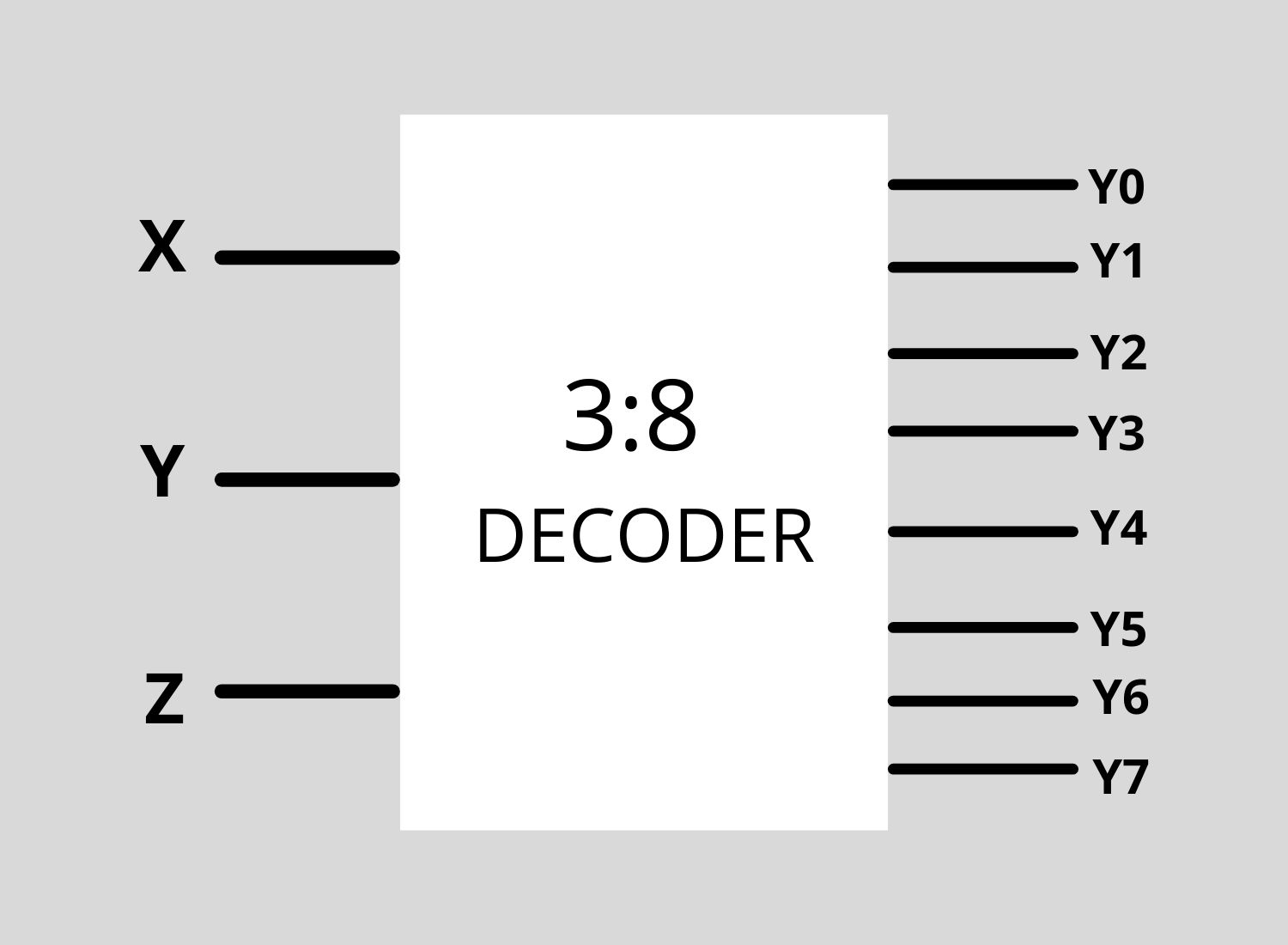3:8 Decoder Block Diagram

An Ideal decoder will set 1 at the given input position. For example, if the Input is 000 then the output's zeroth position (D0) of the output is 1; the rest all outputs will be 0. Likewise, you can observe the truth table below and analyze it.3:8 decoder truth table

Using the truth table, we can construct the Boolean expression to build the logic circuit. And the method used to get Boolean expression is K-Map (Karnaugh map).

We have to apply K-map for every output bit (D0, D1, D2, D4, D5, D6, D7). We get 7 expressions for 7-bit output. Therefore 'n' expressions are obtained for 'n' output lines. Using those obtained expressions, construct the logic circuit.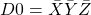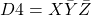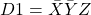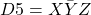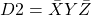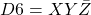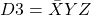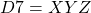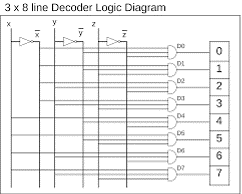3:8 decoder logic diagram

These logic gates are designed to place inside a small chip, as shown below.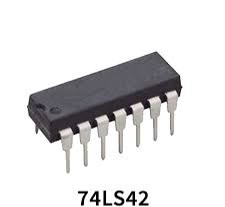IC 74LS42 (Decoder)

## Modified Decoder:

The ideal Decoders can be modified at our convenience. To understand how to alter a decoder and use it as an "Instruction Decoder", we will take an example.

Consider the transmitted signal TxD=01010011(S) and Received Encoded signal RxD=0110. Encoded codes are given in the table below.Encoded codes table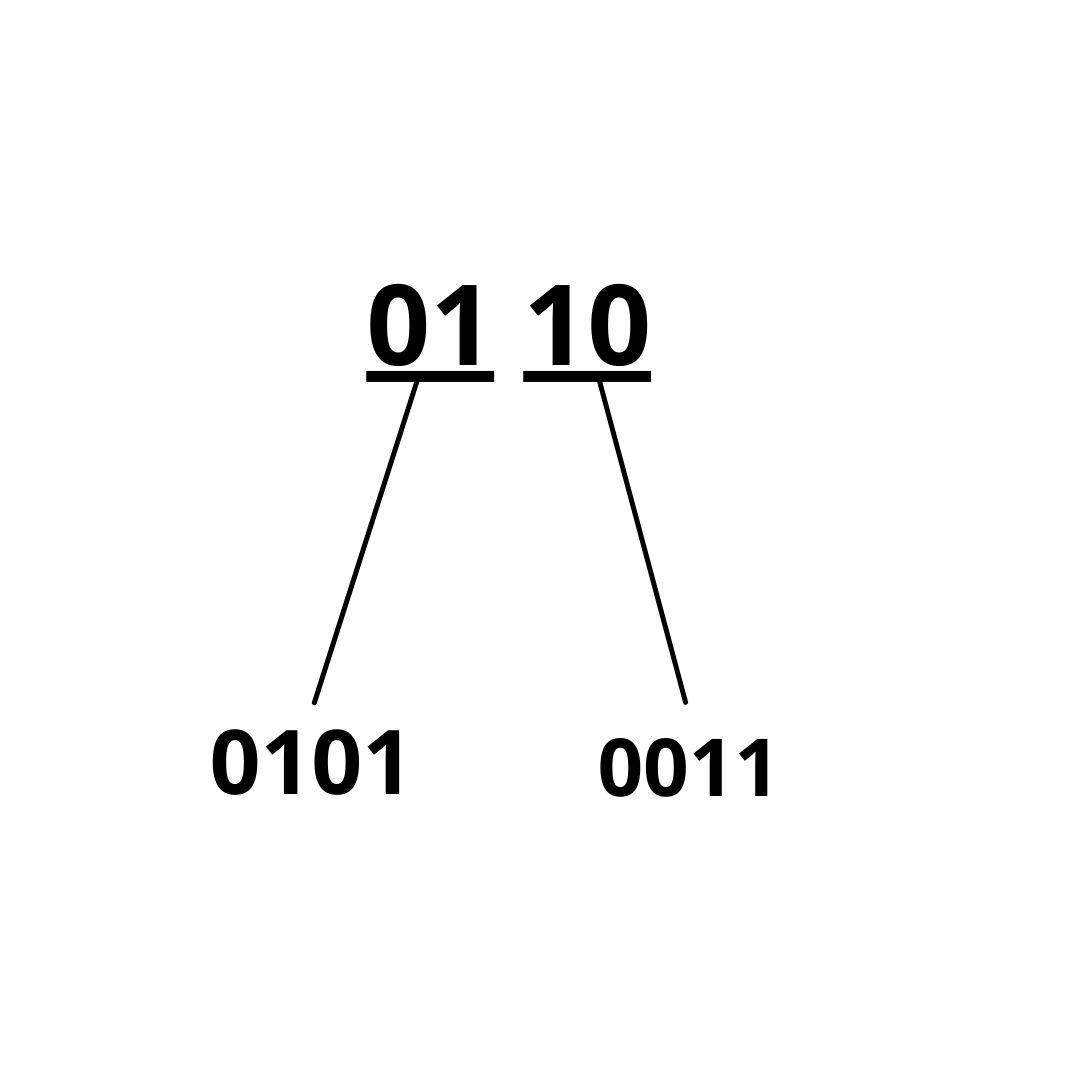Decoding Method

Using the provided Encoded codes. Let's build a Decoder that could decode the information. TxD has 8 bits in the above example, and RxD has 4 bits. Take a ratio of 8:4 that results in 4:2, which is the size of the Decoder to be considered, i.e.2:4 Decoder. Consider 2 bits from received signal 01; see the code table 01 corresponds to 0101 and 10 to 0011. Therefore by joining those, we get 01010011. Yes, We got our original information using Encoded codes. This is the actual logic applied to find a signal encoded message. But to see the decoded message for more than a single encoded message with the same encoded codes. We have to use the same as truth table values for the 2:4 Decoder. We need to get expressions back to our original information logically/generally. Below are the K-map expressions for 4 outputs.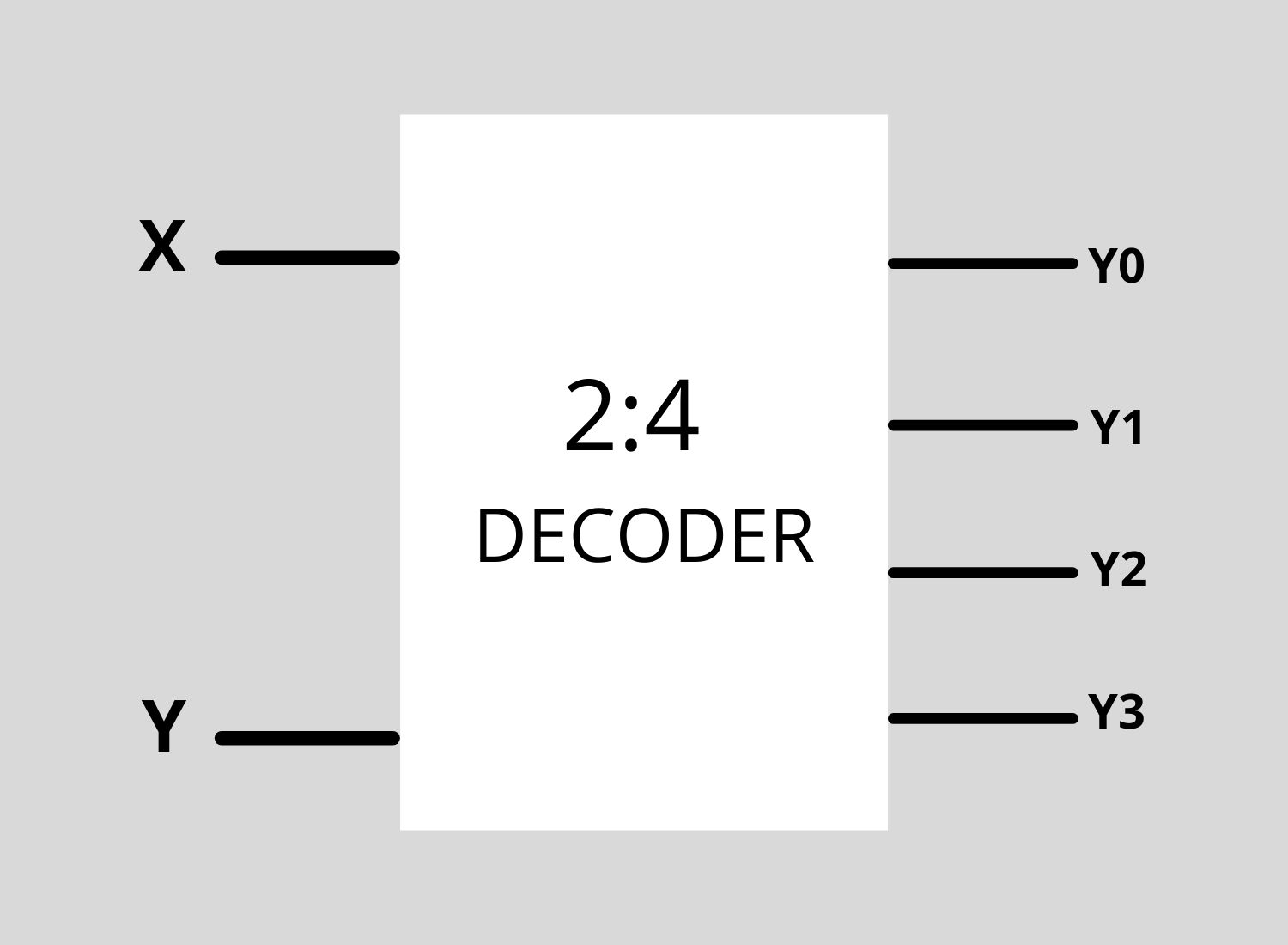2to4 decoder block diagram2:4 Decoder truth table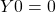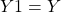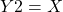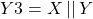## MATLAB Code:

% Author: Sneha G.K

% Topic: Decode Encoded Instruction

%%%%%%%%%%%%%%%%%%%%%%%%%%%%%%%%%%%%%%%%%%%

clc;

clear;

close all;

% This is a code to decode an encoded

% message.

% Take encoded message from user in

% binary form.

TxD=input('Enter the Encoded message:');

% convert the encoded binary message to

% decimal in order use in bit operations.

TxD=bin2dec(string(TxD));

x=zeros(1,4);

% bit rightshift then and with 1 to

% store the LSB bit. This logic is to

% convert the binary value into binary

% vector

for i=1:4

x(i)=bitand(TxD,1);

TxD=bitshift(TxD,-1);

end

k=1;

% To calculate each output bit using

% general equations and store that in

% some temporary variable.

for j=1:2:4

D0=0;

D1=x(j+1);

D2=x(j);

D3=or(x(j),x(j+1));

if k==1

temp=[D0 D1 D2 D3];

end

if k==2

temp1=[D0 D1 D2 D3];

end

k=k+1;

end

% concatenate those temporary vector.

RxD=[temp temp1];

disp(RxD);

## Conclusions:

Decoders are used for Instruction decoding and for Data multiplexing, Data Demultiplexing, Seven Segment displays, and as address decoders for memory and port-mapped I/O.

• A Decoder is an electronic circuit with multiple Input and multiple output signals.
• It has 'n' input lines and 2n output lines.
• Using truth table and K-map method. We can get a decoder.
• Use the obtained expression in MATLAB code and start MATLABing.

Did you find some helpful content from our video or article and now looking for its code, model, or application? You can purchase the specific Title, if available, and instantly get the download link.

Thank you for reading this blog. Do share this blog if you found it helpful. If you have any queries, post them in the comments or contact us by emailing your questions to [email protected]. Follow us on LinkedIn Facebook, and Subscribe to our YouTube Channel. If you find any bug or error on this or any other page on our website, please inform us & we will correct it.

If you are looking for free help, you can post your comment below & wait for any community member to respond, which is not guaranteed. You can book Expert Help, a paid service, and get assistance in your requirement. If your timeline allows, we recommend you book the Research Assistance plan. If you want to get trained in MATLAB or Simulink, you may join one of our training modules.

If you are ready for the paid service, share your requirement with necessary attachments & inform us about any Service preference along with the timeline. Once evaluated, we will revert to you with more details and the next suggested step.

Education is our future. MATLAB is our feature. Happy MATLABing!

#### Sneha

I am Sneha G.K . I am an Electronic and Communication Engineering Student. I am a passionate Programmer and a researcher too. I enjoy exploring new things everyday and experimenting it.

{"email":"Email address invalid","url":"Website address invalid","required":"Required field missing"}

#### Connect with MATLAB Helper ®

Join Community of MATLAB Enthusiasts: Facebook Group, Telegram, LinkedIn Group

Use Website Chat or WhatsApp at +91-8104622179

Watch our Latest Video

MATLAB & Simulink Experts